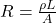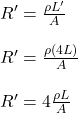## First term / second mid Exam [1433/ 1434] Q12. A conductor of radius I, length and resistivity p has resistance R. What is the new resistanc

Question

First term / second mid Exam [1433/ 1434] Q12. A conductor of radius I, length and resistivity p has resistance R. What is the new resistance if it is stretched to 4 times its original length?

in progress 0
6 months 2021-08-22T05:41:03+00:00 1 Answers 4 views 0

## Answers ( )

R’ = 4R

Hence, the resistance increases to four times its initial value as well.

Explanation:

The resistance of a conductor in terms of its physical dimensions can be given by the following formula:_______ equation (1)

where,

R = Resistance of the conductor

ρ = resistivity of the material of the conductor

L = Length of the conductor

A = Cross-sectional Area of the conductor

Now, if the length of the conductor is increased to four times, keeping other values constant, then:using equation (1):

R’ = 4R

Hence, the resistance increases to four times its initial value as well.